# SAT Math Multiple Choice Question 974: Answer and Explanation

### Test Information

Question: 974

10.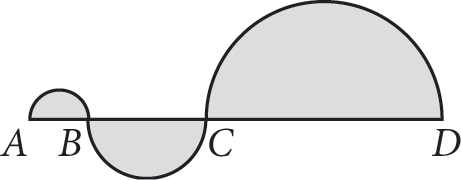Each of the three shaded regions above is a semicircle. If AB = 4, BC = 2AB, and CD = 2BC, what is the area of the entire shaded region?

• A. 28π
• B. 42π
• C. 84π
• D. 96π

Strategic Advice: Use the only diameter given (AB = 4) to determine the other two diameters. Once there, use A = πr2 to find the area of each. Remember to multiply each area by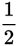to account for the fact that you have semicircles, not whole circles.
Getting to the Answer: The diameter of semicircle AB is given as 4, so its radius is 2. Therefore, the area of semicircle AB is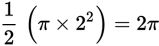. If BC = 2AB, then BC = 8, and the radius of semicircle BC is 4. Its area, therefore, is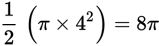. Similarly, because CD = 2BC, CD must equal 16, and the radius of semicircle CD is 8, giving it an area of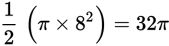. Add the three areas together to get 2π + 8π + 32π = 42π, which matches (B).